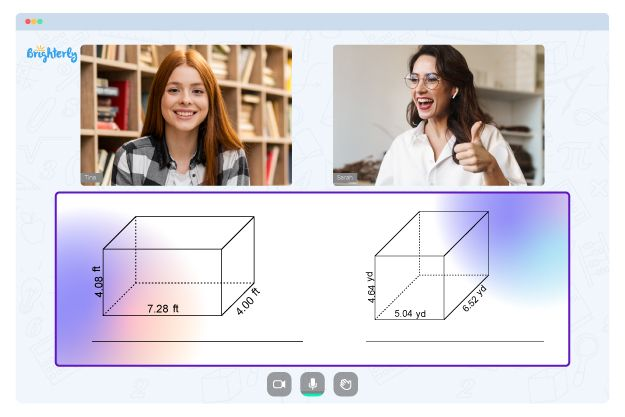# Scale Factor Worksheets

These scale factor worksheets are designed to help students learn about scale factors. With this scale factor problems worksheet, kids can learn scale drawing and geometry drawings. They will also be able to solve problems that involve shapes. These worksheets are appropriate for kids in the 7th grade.

## What is the Scale Factor?

A scale factor is a number (the conversion factor) that is used to change a figure’s size without altering its shape. You can either use it to decrease or increase the size of an object.

However, scale factor can only be calculated if the dimensions of the dilated figure and original figure are known. With scale factor, you can draw the reduced or enlarged shape of any figure. You can also use it to find the area, volume, or length of a reduced or enlarged figure.

Math for Kids

Is Your Child Struggling With Math?
1:1 Online Math Tutoring## Benefits of Scale Factor Worksheet

This scale factor with answers worksheet PDF will help students understand how measurement works. This is especially important as measurement is one of the major foundational concepts in Math. This scale factor worksheet PDF comes with problems and answers that will help your child learn how to apply the concept of measurement to real world problems.### Scale Factor Worksheets PDF

Scale Factor Worksheet### Scale Factor Worksheets PDF

Scale Factor Worksheet With Answers PDF### Scale Factor Worksheets PDF

Scale Factor Worksheet PDF### Scale Factor Worksheets PDF

Scale Factor Problems Worksheet

With the scale factor worksheet 7th grade PDF with answers, students will also learn how to reduce or enlarge scale drawing of a given factor. This means they will be able to reduce or increase the shape of an object. They will also be able to find missing information in a figure.

### More Worksheets

Need help with Geometry?• Does your child struggle with mastering geometry lessons?
• Try learning with an online tutor.

Is your child having difficulties grasping of geometry? An online tutor could provide the necessary help.